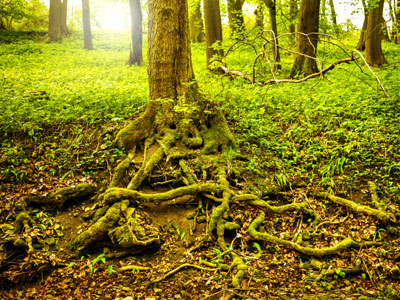How good are you at roots? That is, mathematical roots not tree roots!

# Level 5-6 Numbers - Roots

So, KS3 Maths has made you familiar with square numbers and cube numbers. They are powers denoted by a 2 or a 3 above and to the right of another number. For example, 3 squared is 32 and 3 cubed is 33. But there is another side to squares and cubes - roots.

A number multiplied by itself creates its own square. The original number is called the square root of the new number. For example, the square root of 9 is 3 because 3 x 3 = 9. It's similar with cube roots. A number multiplied by itself twice creates a cube number. The original number is the cube root of this. For example, the cube root of 64 is 4 because 4 x 4 x 4 = 64. It's not easy to work out square or cube roots. It usually involves a great deal of trial and error - or a calculator!

It would be great if you could memorise the square and cube values of lower numbers. That would help you a lot but might take a considerable amount of time. In the meanwhile, try this quiz to get some practise with square and cube roots..

1.
What is the square root of 54?
15
25
125
250
To find the square root of a power number you divide the index by 2. √(54) = 52
2.
A square of area 169 cm2 has side length .......
43 cm
33 cm
23 cm
13 cm
√169 = 13
3.
A cube of volume 1,000 cm3 has side length .......
1 cm
10 cm
100 cm
1 m
∛1,000 = 10
4.
The square root of 425 lies between .......
19 and 20
20 and 21
21 and 22
23 and 24
202 = 400; 212 = 441. √425 = 20.61552813.......
5.
The cube root of 216 is .......
6
16
26
36
Since 162 = 256, 163 must be bigger and 263 or 363 will be bigger still, so 6 is the only sensible answer
6.
The square with the same area as a rectangle measuring 9 cm by 16 cm has side length .......
6 cm
9 cm
12 cm
15 cm
9 x 16 = 144. √144 = 12
7.
What is the cube root of 79?
49
147
343
560
To find the cube root of a power number you divide the index by 3. ∛(79) = 73
8.
Which of these is not a way of writing the square root of 625?
25
52
√125
6251⁄5
The square root of 125 is 11.18 to two decimal places.
9.
What is the total surface area of a cube which has a volume of 729 cm3?
54 cm2
81 cm2
360 cm2
486 cm2
∛729 = 9 so each square face has an area of 81 cm2. A cube has six square faces; 6 x 81 = 486
10.
What is the square root of 64 x 144?
96
72
60
48
The square root of a product is the product of the square roots. √64 x √144 = 8 x 12